## Examples

In formaldehyde, the carbon is the central atom, so we can draw a skeletal structure: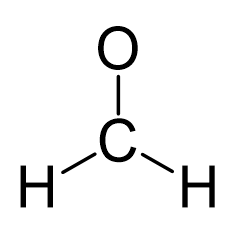There is a total of 4 + 2×1 + 6 = 12 electrons, and 6 are already used for making the bond. The remaining 6 go to oxygen as it is the most electronegative atom.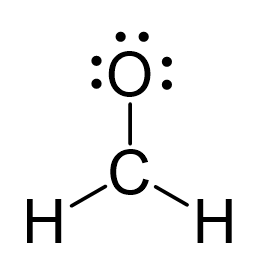Because the carbon lacks an octet, we use one lone pair from the oxygen to make a π bond with the carbon, and thus, a double bond is formed.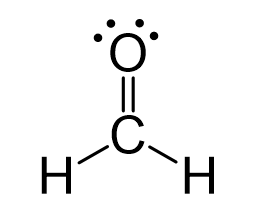The central atom has three bonds and no lone pairs, therefore, both the electron and molecular geometries are trigonal planar.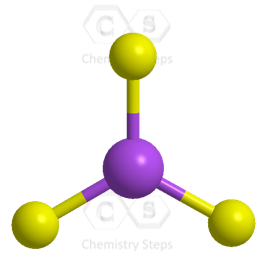For a trigonal planar geometry, the expected bond angles are 120o, however, because the three groups are not identical, the bond angles will not be as in idealized geometry.

In general, remember that oxygens on terminal positions are always going to have 2 bonds and 2 lone pairs of electrons unless there is a formal charge on them.

Knowing these patterns for bonding, lone pairs, and formal charges will make these types of exercises a lot easier.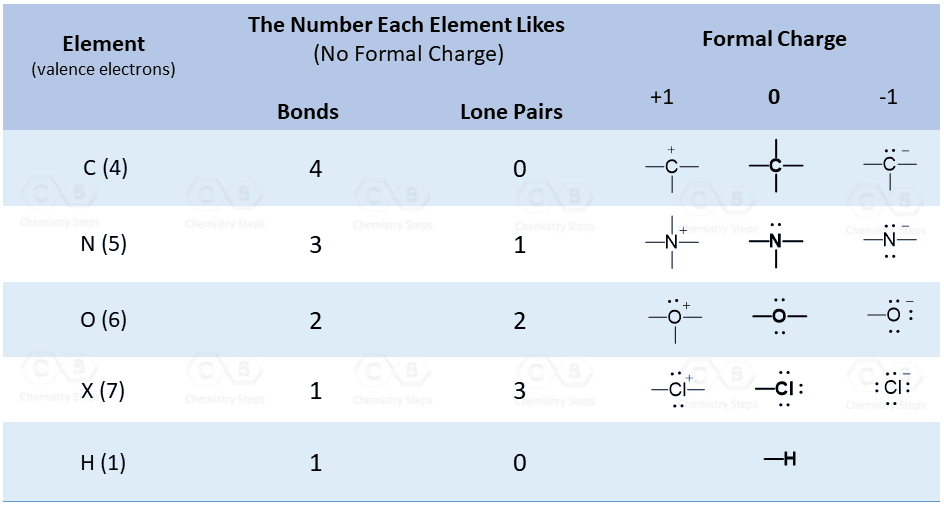The steric number (the sum of the number of the atoms and lone pairs) of the carbon is 3 which corresponds to sp2-hybridization.

Check Also